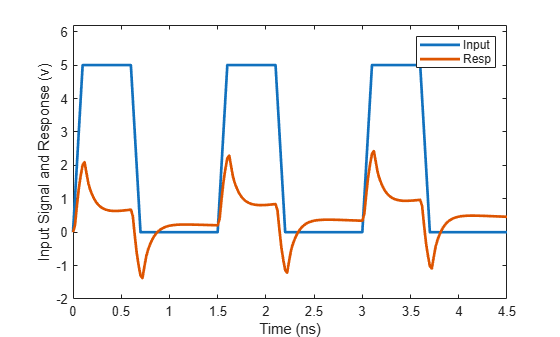# pwlresp

Calculate time response of piecewise linear input signal

Since R2021a

## Syntax

``[tran,t] = pwlresp(h,signalTime,signalValue,tsim)``
``[tran,t] = pwlresp(h,signalTime,signalValue,tsim,tper)``
``[tran,t] = pwlresp(h,signalTime,signalValue,tsim,tper,flag)``

## Description

````[tran,t] = pwlresp(h,signalTime,signalValue,tsim)` computes the time response, `tran`, and time vector, `t`, of a piecewise linear input signal. The time response is calculated for a `rational` or `rfmodel.rational` object, `h`, using the signal parameters, `signalTime` and `signalVlaue`, over the simulation time, `tsim`. ```

example

````[tran,t] = pwlresp(h,signalTime,signalValue,tsim,tper)` computes the time response for the period of the input signal, `tper`. ```
````[tran,t] = pwlresp(h,signalTime,signalValue,tsim,tper,flag)` computes the time response across a set of time points that speed up the time response computation. NoteUse the `flag` input argument only for periodic signals. When you use the `flag` input, the first element of `tsim` vector should be `0`. ```

## Examples

collapse all

Calculate the transient output waveform when a piecewise linear input signal is applied to a system described using a `rational` object.

Perform Rational Fit

Read the specified S2P data file.

```S = sparameters('passive.s2p'); freq = S.Frequencies;```

Convert the S-parameters of the two-port network to a transfer function and fit to a `rational` object.

```tf_data = s2tf(S); h = rational(freq,tf_data);```

Define Parameters of Periodic Input Signal

Define the parameters of a periodic input signal over its first period, `tper`.

```signalTime = [0,0.1,0.6,0.7,1.5]*1e-9; signalValue = [0,5,5,0,0]; tper = 1.5e-9;```

Calculate and Plot Transient Response

Calculate the transient response over three time periods for the simulation time interval, `ts`.

```ts = 2e-11; tsim = 0:ts:3*tper; [tran,t] = pwlresp(h,signalTime,signalValue,tsim,tper);```

Plot the output response and the periodic input signal.

```vin = repmat(signalValue,1,3); tin = [signalTime,signalTime+tper,signalTime+2*tper]; figure plot(tin*1e9,vin,t*1e9,tran,'LineWidth',2) axis([0 4.5 -2 6.2]); xlabel('Time (ns)'); ylabel('Input Signal and Response (v)'); legend('Input','Resp');```## Input Arguments

collapse all

Rational fit object, specified as either an `rfmodel.rational` or a `rational` object.

Input signal time, specified as a vector of positive integers in seconds.

Input signal amplitude, specified as a vector of integers. The signal amplitude corresponds to the input signal time specified in `signalTime`.

Note

For a periodic input signal, specify the `signalValue` over only to the first period of the signal.

Simulation time, specified as a vector of positive integers in seconds.

Period of the input signal, specified as a positive scalar in seconds.

Flag to speed up the time response computation, specified as `'Rapid'`. Use the `flag` argument for the function to rapidly compute the time response.

Note

When you specify `flag` as an input argument to this function, the time vector, `t` corresponding to the output response ,`tran`, might be different from the simulation time `tsim` specified.

## Output Arguments

collapse all

Output response of a piecewise linear input signal, returned as a nonnegative vector.

Time vector corresponding to the output response, `tran`, returned as a nonnegative vector.

## Version History

Introduced in R2021a